> > Dices Test

# Dices Test

Dices test and cubes test hold a very special place in reasoning ability because these questions rely mainly on your intellectual and visualizing capacity. It is important that you have enough practice and experience of these questions beforehand. We will help you with some of the concepts, tricks, and understanding of dices test questions.

### Suggested VideosColoured Cube ISequence of Occurence of Events in a ProcessBlood Relations Introduction## Dices Test

Before knowing about Dices Test let’s know some basics about dice. There are generally two types of dice, open and base dice. Base dice further divide into two types, standard and ordinary dice. When the numbers in the two dice do not match each other they are called a standard dice. While if one or more than one number matches between two dice than it is called ordinary dice.Cubes and Dices Test

### Points To Remember

To solve dices test questions it is important that you learn some rules first. Here are some of the important rules that can help you during the exams:

• When two dice have same surface and one of the number/element is common in both the dices than the remaining surfaces of the dice will have opposite elements in them.
• For example, if two dice have number 4 as a common element and 3,5 on one dice and 1,2 on the surface of the other dice then both 3,5 and 1,2 are opposite to each other.
• If there are two numbers in the dices that are same irrespective of their position on the dice then the third number left in both the dices will be opposite to each other.
• When there is one element common to both the dices which have different surface areas then rotate the dices in a clockwise direction so that you can find out the opposite surface.

When you open the dice this is how it will look like:### Rules Related To Open Dices

Some of the rules related to the open dices based on the above figure are:

– 5 and 6 are always opposite to each other. This is true for any type of open dice whether it be 2 and 6, 2 and 6 or 3 and 4.

– 1 and 3 do not touch each other so they are in the opposite direction.

– Similarly, surfaces of 2 and 4 also do not touch each other and therefore they are also in opposite direction.

Now we will provide you with some of the solved examples so you can have a better understanding of the topic.

## Expected Questions

Q 1. In the figures below four different dices are shown. When the dice is as shown in the figure than what must be the value at the bottom face of the dice?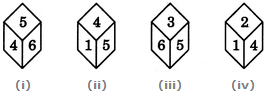1. 2                  B. 1

3. 4                 D. 5

Ans: For these type of questions look for the number that is common in the figure. In this question, this number is 4. And from the figure 1, 2, and 4 you can see four numbers that are adjacent to the number 4. Therefore the remaining the number 3 will lie opposite to 4 and vice versa. While in the only figure without 4 i.e. the figure 3 opposite to the number 3 will be 4. Thus the correct option is (3)

Q 2. If in the below figures the total value of the opposite value is always seven than which figure is the correct one?1. Figure 1                    B. Figure 2

3. Figure 3                   D. Figure 4

Ans: In figure 1; 1, 2, and 6 are there on the dice the remaining 3, 4, and 5 cannot be arranged in any way which can make the opposite total to 7. In figure 3; 3, 4, and 6 are given. The remaining 1, 2, and 5 cannot be arranged to make the sum of all the opposite faces to 7. In figure 4, only two opposite faces can make the sum 7. The only figure left is the option (2) wherein you can use the remaining values and the sum of the opposite faces will be 7.

Q 3. Which number in the below figures will be opposite to the number 6?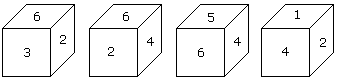A. 1                     B. 2

C. 3                    D. 4

Ans: From the figure 1, 2, and 3 the numbers that are adjacent to 6 can be found out. They are 2, 3, 4, and 5. The only number that is left is 1 which is opposite to 6. Thus the correct option is (1).

## Practice Questions

Q 1. Given in the figure are two dices. When two dots are at the bottom, how many dots will be at the top? (10)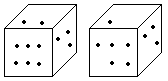1. 4                       2. 3

3. 2                      4. 1

Q 2. The position of two dices is shown in the figure. If one dot is at the bottom than how many dots will be at the top of the dice?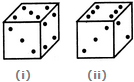1. 1                        2. 2

3. 3                       4. 4

Q 3. In the below figure, four dices are positioned. What will be the opposite face of the one that has 3 dots?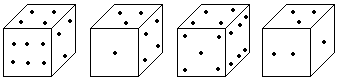1. 3                        2. 4

3. 2                       4. 5

Q 4. Based on the position of alphabets in the cube, what letter will be the opposite of A?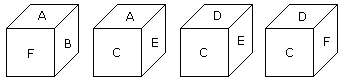1. B                      2. D

3. C                     4. F

Share with friends

## Customize your course in 30 seconds

##### Which class are you in?
5th
6th
7th
8th
9th
10th
11th
12th
Get ready for all-new Live Classes!
Now learn Live with India's best teachers. Join courses with the best schedule and enjoy fun and interactive classes.Ashhar Firdausi
IIT Roorkee
BiologyDr. Nazma Shaik
VTU
ChemistryGaurav Tiwari
APJAKTU
Physics
Get Started

## Browse

##### Cubes and Dices TestSubscribe
Notify of

## Question Mark?

Have a doubt at 3 am? Our experts are available 24x7. Connect with a tutor instantly and get your concepts cleared in less than 3 steps.